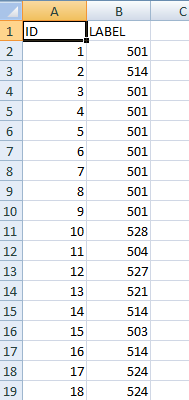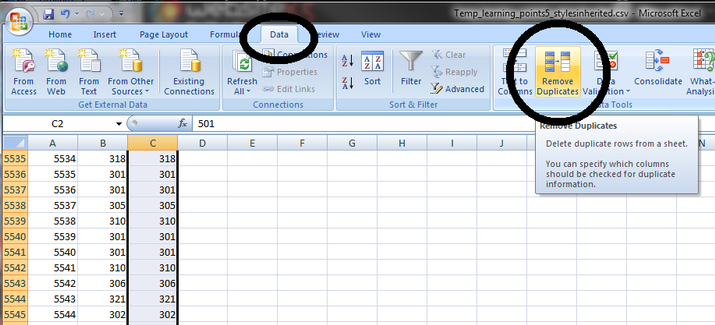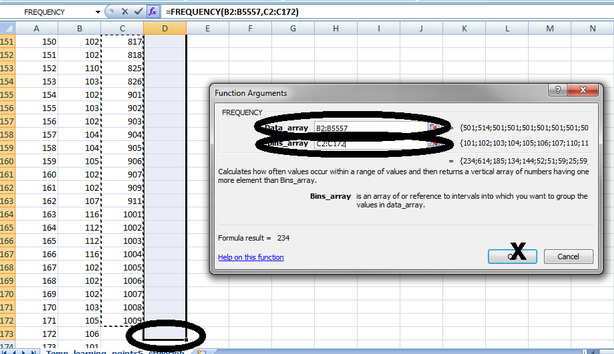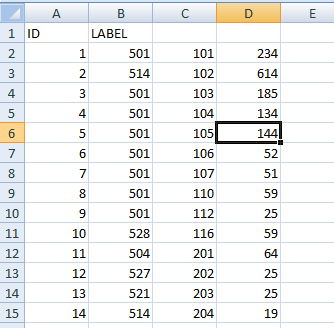# A20.2 Excel: Frequency - LUPMISManual

Go to content

Annexes 11-20 > A20. Various IT advices

A20.2 Excel: Frequency

Level of expertise required for this Chapter: Intermediate

For a GIS crosstabulation to produce statistics how many points occur in particular areas, you should be familiar with the Excel 'Frequency' command. A very similar task can be carried out with the conditional sum tool of Excel (explained in Annex 20.5) for overlay and sumation of areas.

As explained in Chapter 5.1.9 ('Frequency analysis of points'), you can overlay points on polygons and create a table, which lists all points with their respective polygon (District number). You want to know how many points are in each polygon (District), e.g. in example below, how many rows with 501, 514 etc.1. Copy the data of column B to a new column (e.g. C), then select this data range, and
Data > Remove Duplicates .2.
Sort this particular data set from smallest to largest: Select this data range, and Data > Sort.

3. Select a range next to the previous (e.g. in column D), but it should be one row longer than the previous, and call the Function command through
Insert Function (fx) > Type: Frequency > Go > Data_array: Select the data range with the original polygon numbers (e.g. B) > Bins_array: Select the data range, just sorted (e.g. C) > Do not press Enter or OK, but Ctrl-Shft-Enter !There it is: The new column (e.g. D) list the occurrencies on each number in the column left (e.g. C). In the example, there are 234 occurrencies (masts) in District 101, etc.4. To work with the individual figures, you still have to
Copy > Paste special > Values.